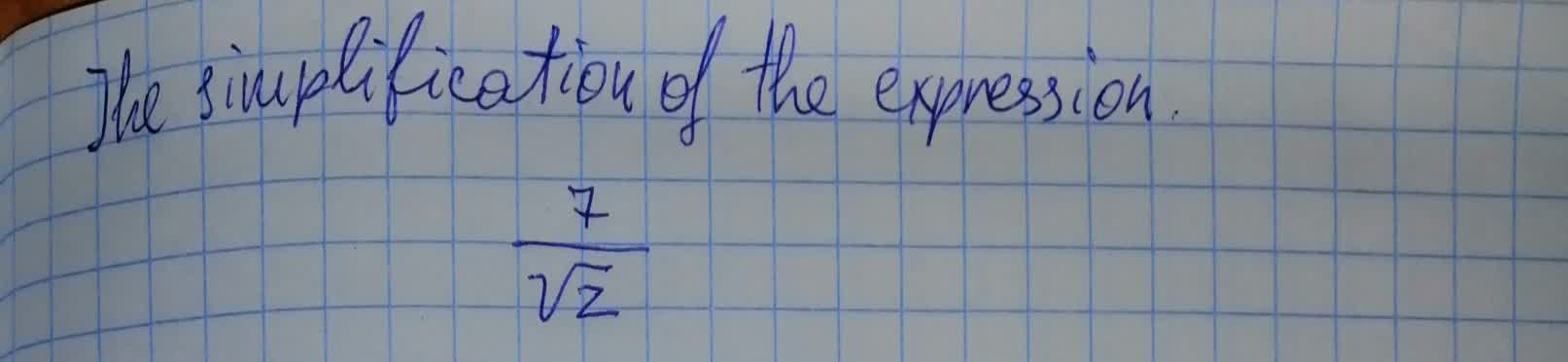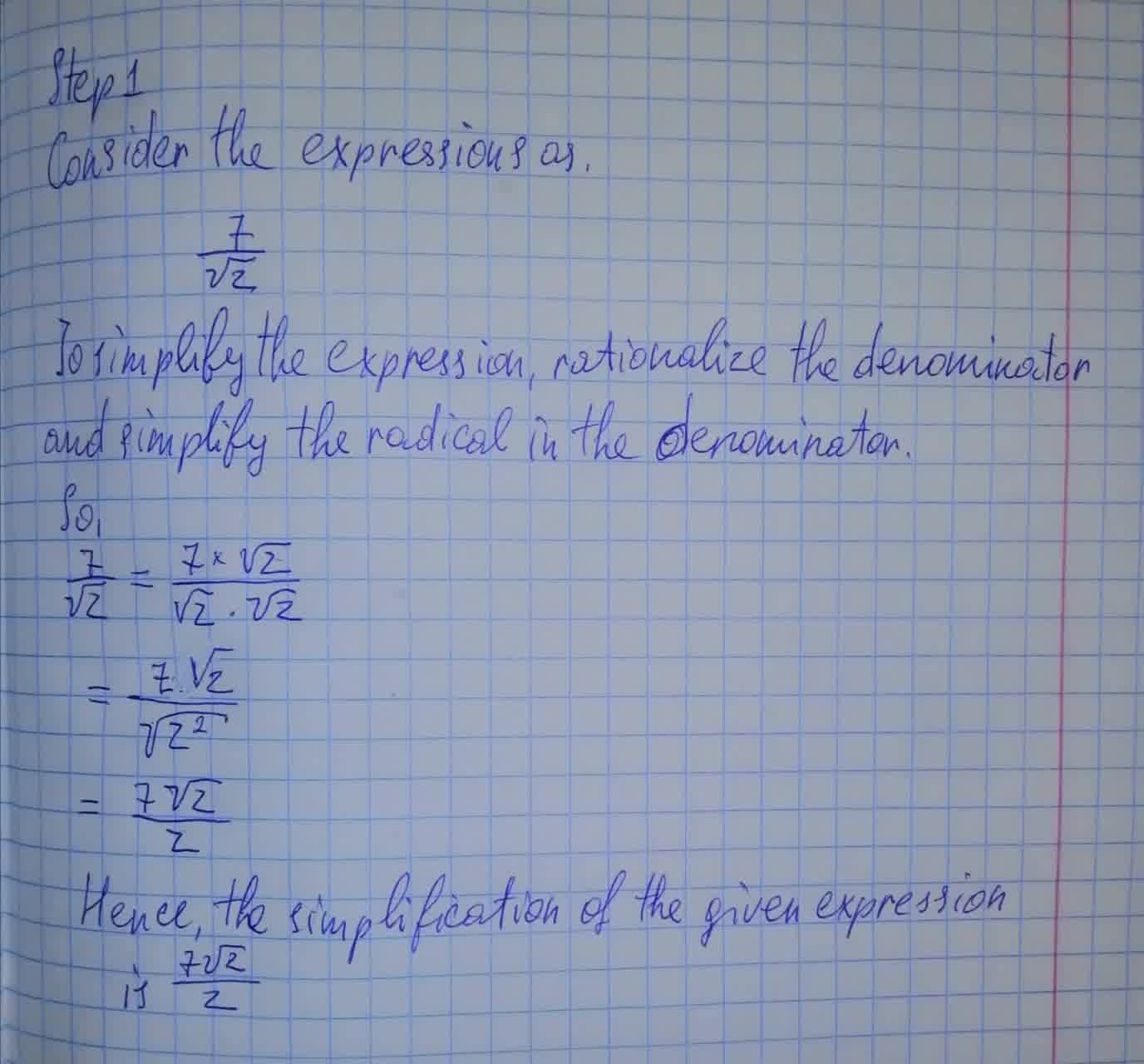Ayaana Buck

2021-08-08

To calculate: The simplification of the expression $\frac{7}{\sqrt{z}}$Laith Petty

To solve:2021-12-24

Step 1

Consider the expressions as,

$\frac{7}{\sqrt{z}}$

To simplify the expression, rationalise the denomination and simplify the radical in the denomination

So,

$\frac{7}{\sqrt{z}}=\frac{7×\sqrt{z}}{\sqrt{z}\cdot \sqrt{z}}$

$=\frac{7\sqrt{z}}{\sqrt{{z}^{2}}}$

$=\frac{7\sqrt{z}}{z}$

Hence, the simplification of the given expression is $\frac{7\sqrt{z}}{z}$

Do you have a similar question?